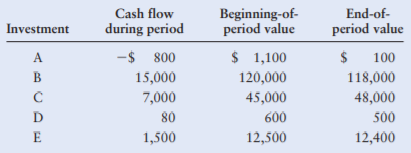### Create an Account

Home / Questions / Return calculations For each of the investments shown in the following table calculate the...

# Return calculations For each of the investments shown in the following table calculate the rate of return earned over the unspecified time period

Return calculations For each of the investments shown in the following table, calculate the rate of return earned over the unspecified time period.Jun 02 2021 View more View LessGet Solution